« Exercise 4.9.5 Exercise 4.9.7 »

Exercise 4.9.6

Given : Covering load gεπ=1.0 kN/m2, live load q= 10.0 kN/m2, slab thickness h=140 mm.

Question: Determine bending moments of the slabs in the figure having three spans in x direction and practically infinite number of spans in y direction.

Solution:

The design loads to be used for loading the slabs are gd and pd=1.35g+1.50q= g+(0.35g+1.50q) which is analyzed to: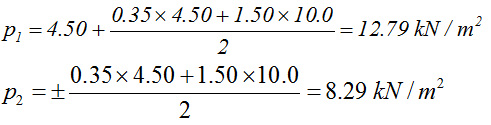Static analysis

Moments are determined by means of the technique described in §4.6.4. According to that, the response of the slabs is determined by the particular loadings applied. Thus, while there are actually two slab types, one with four fixed edges and one with three fixed and one free edge, two extra slab types are created: one with four free edges and one with three free and one fixed edge (as illustrated in the figures analyzing the loadings). For ε=ly/lx=4.0/4.5=0.89: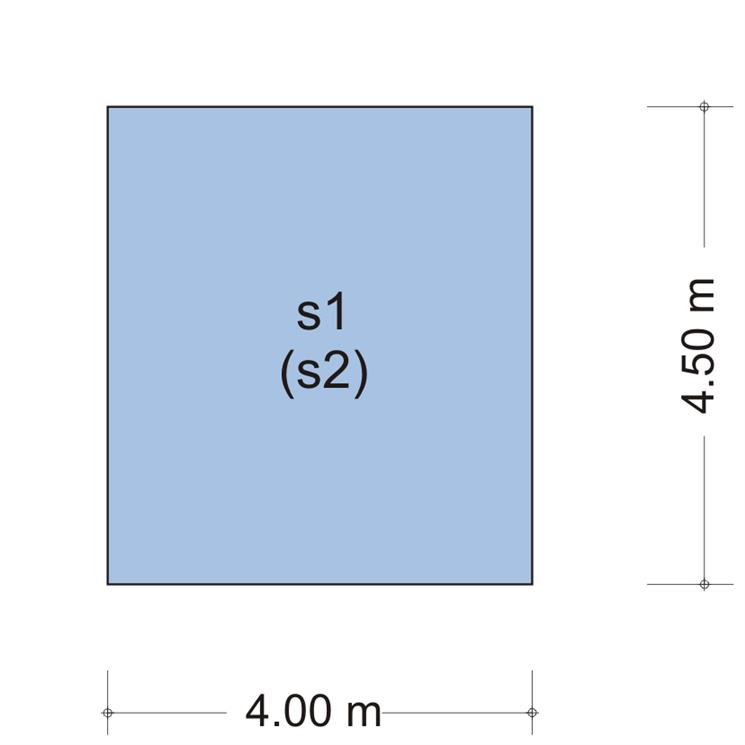Figure 4.9.6-2 Figure 4.9.6-2 For type '1' slab from table b5.1 for ε=ly/lx=4.5/4.0=1.125 → kx,1=0.615, ky,1=0.385, vx,1=vy,1=0.595 → p2x=0.615x8.29=5.10 kN/m, p2y=0.385x8.29=3.19 kN/m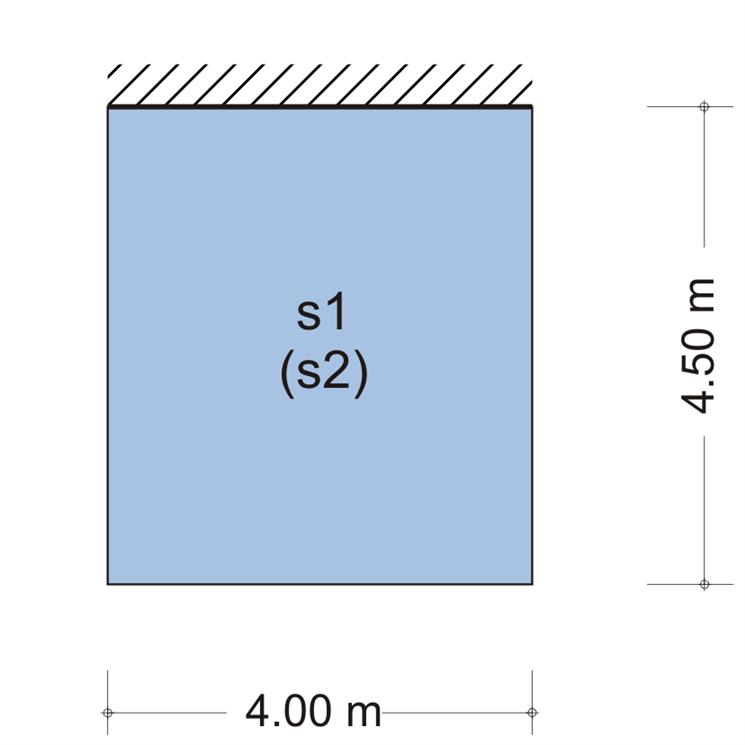Figure 4.9.6-3 Figure 4.9.6-3 For type '2' slab from table b5.2 for ε=ly/lx=4.5/4.0=1.125 → kx,1=0.391, ky,1=0.609, vx,1=0.744, vy,1=0.639 → p2x=0.391x8.29=3.24 kN/m, p2y=0.609x8.29=5.05 kN/m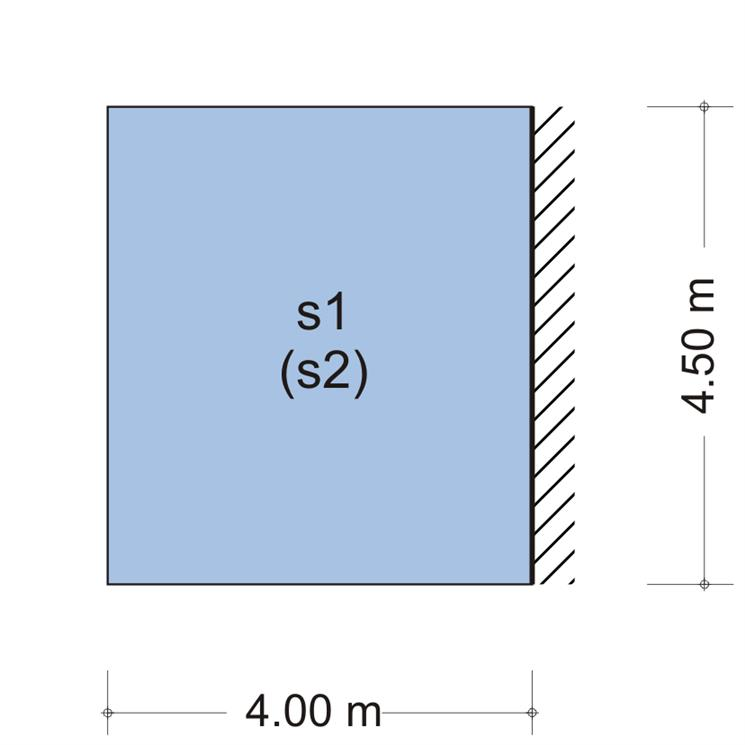Figure 4.9.6-4 Figure 4.9.6-4 For type '2' slab from table b5.2 for ε=ly/lx=4.0/4.5=0.89 → kx,1=0.799, ky,1=0.201, vx,1=0.703, vy,1=0.789 → p2x=0.799x8.29=6.62 kN/m, p2y=0.201x8.29=1.67 kN/m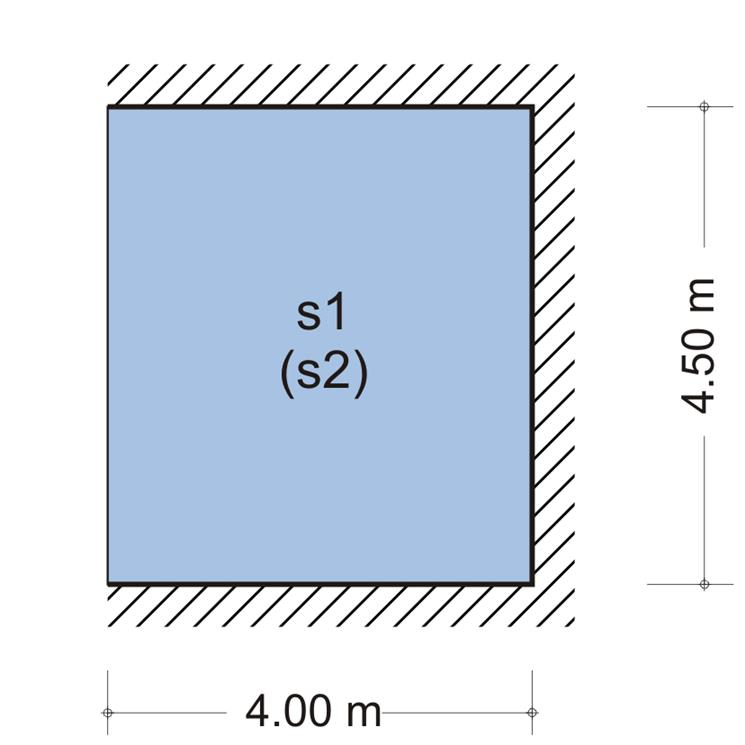Figure 4.9.6-5 Figure 4.9.6-5 For type '5' slab from table b5.5 for ε=ly/lx=4.5/4.0=1.125 → kx,1=0.445, ky,1=0.555, vx,1= 0.835, vy,1=0.805 → p1x=0.445x12.79=5.69 kN/m, p1y=0.555x12.79=7.10 kN/m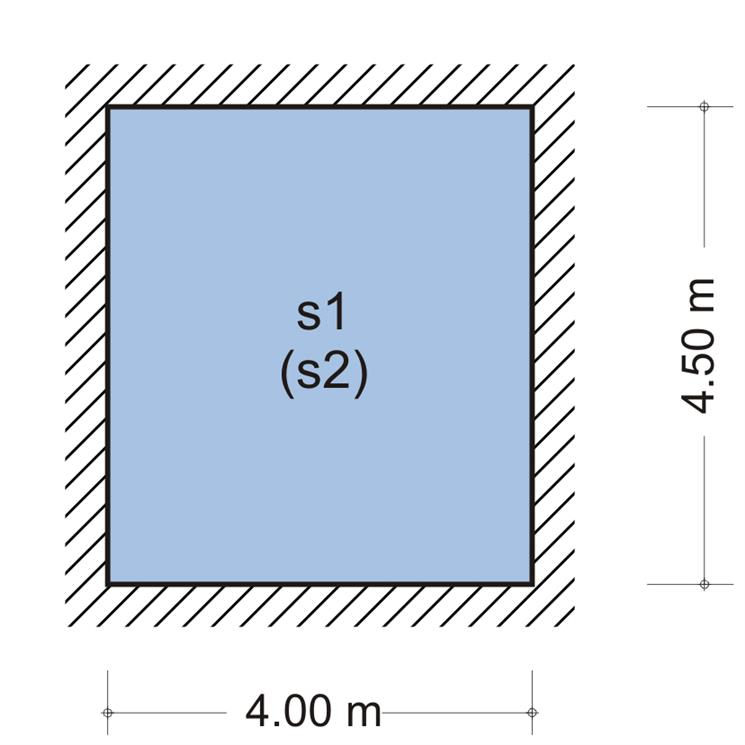Figure 4.9.6-6 Figure 4.9.6-6 For type '6' slab from table b5.6 for ε=ly/lx=4.5/4.0=1.125 → kx,1=0.615, ky,1=0.385, vx,1=vy,1=0.865 → p1x=0.385x12.79=4.92 kN/m, p1y=0.615x12.79=7.87 kN/m

minMs1-s2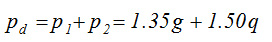≡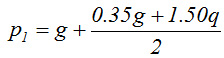+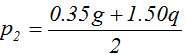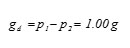≡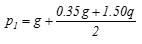-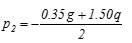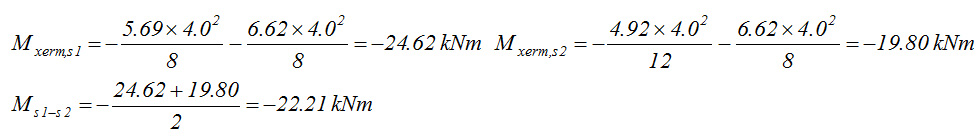minMs1-s1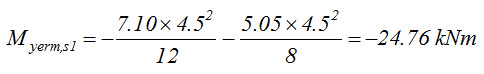minMs2-s2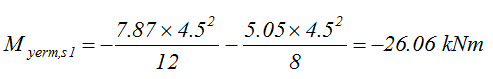maxMs1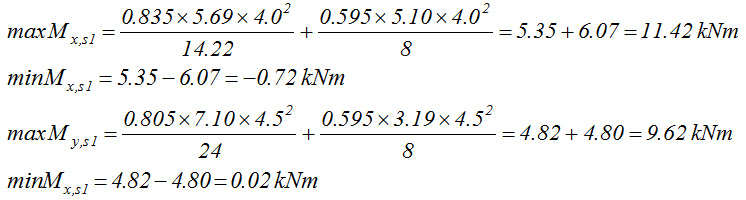maxMs2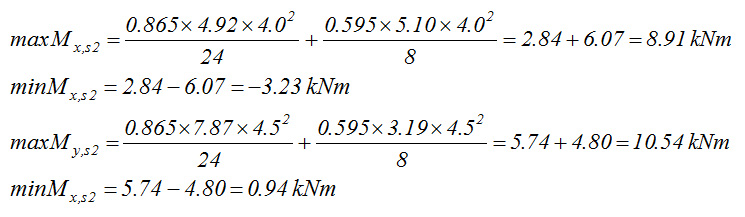« Exercise 4.9.5 Exercise 4.9.7 »